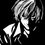# This sum will give you nightmares!!!

In my entry to Troll King Contest, I posed the following problem -

Evaluate

$\sum_{a=0}^\infty \sum_{b=0}^\infty \sum_{c=0}^a \sum_{d=0}^b \sum_{e=0}^d {n+a \choose a} {b-e \choose d-e} {a \choose c} {b \choose e} (-1)^{b+c} n^e c^{d-e}$

Let's see who can dare to attempt it.

I'll post my solution, once the troll contest ends.

Also notice that, this problem inspite of an entry in Toll King Contest serves another purpose of explaining a little bit about multiple summations to my friends who requested it.

[Hint for solving that troll problem : After reading the complete problem, ask yourself that do I really need to troll with the above summation]Note by Kishlaya Jaiswal
4 years, 11 months ago

This discussion board is a place to discuss our Daily Challenges and the math and science related to those challenges. Explanations are more than just a solution — they should explain the steps and thinking strategies that you used to obtain the solution. Comments should further the discussion of math and science.

When posting on Brilliant:

• Use the emojis to react to an explanation, whether you're congratulating a job well done , or just really confused .
• Ask specific questions about the challenge or the steps in somebody's explanation. Well-posed questions can add a lot to the discussion, but posting "I don't understand!" doesn't help anyone.
• Try to contribute something new to the discussion, whether it is an extension, generalization or other idea related to the challenge.

MarkdownAppears as
*italics* or _italics_ italics
**bold** or __bold__ bold
- bulleted- list
• bulleted
• list
1. numbered2. list
1. numbered
2. list
Note: you must add a full line of space before and after lists for them to show up correctly
paragraph 1paragraph 2

paragraph 1

paragraph 2

[example link](https://brilliant.org)example link
> This is a quote
This is a quote
    # I indented these lines
# 4 spaces, and now they show
# up as a code block.

print "hello world"
# I indented these lines
# 4 spaces, and now they show
# up as a code block.

print "hello world"
MathAppears as
Remember to wrap math in $$ ... $$ or $ ... $ to ensure proper formatting.
2 \times 3 $2 \times 3$
2^{34} $2^{34}$
a_{i-1} $a_{i-1}$
\frac{2}{3} $\frac{2}{3}$
\sqrt{2} $\sqrt{2}$
\sum_{i=1}^3 $\sum_{i=1}^3$
\sin \theta $\sin \theta$
\boxed{123} $\boxed{123}$

Sort by:

Answer as per me is $1$

Since the sum is large and solution long, so i shall write only the usable parts of the sum while writing it, please bear with me

Let us first consider the summation of 'e' terms

it can be shown with some manipulation that (as i have told, the other terms have been ommitted for clarity)

$\sum { (\begin{matrix} b-e \\ d-e \end{matrix})(\begin{matrix} b \\ e \end{matrix})({ \frac { n }{ c } }^{ e }) } \quad =\quad \quad \sum { (\begin{matrix} b \\ d \end{matrix})(\begin{matrix} d \\ e \end{matrix})({ \frac { n }{ c } }^{ e }) } =(\begin{matrix} b \\ d \end{matrix})(1+\frac { n }{ c } )^{ d }$

where the summation is over 'e'

now , let us consider the sum over 'd'

we have the 'd' having terms as (now)

$\sum { (\begin{matrix} b \\ d \end{matrix})(1+\frac { n }{ c } )^{ d }{ c }^{ d } } \quad =\quad \sum { (\begin{matrix} b \\ d \end{matrix})(n+c)^{ d } } =(1+n+c)^{ b }$

now, since the summation over 'b' is not related to that over 'c' in any way, so we perform the sum over 'b' first to write

consider all 'b' terms

$\sum { (-1)^{ b } } (1+n+c)^{ b }\quad =\quad \frac { 1 }{ 2+n+c } \quad (from\quad taylor\quad series\quad of\quad \frac { 1 }{ 1+x } \quad at\quad x=\quad 1+n+c\quad )$

where summation was over 'b'

now , we have to sum over 'c' to 'a' first and then 'a' to $\infty$

so consider all 'c' terms presently there

$\sum { \frac { (-1)^{ c } }{ 2+n+c } } (\begin{matrix} a \\ c \end{matrix})\quad$

to solve it , consider

$(1-x)^{ a }\quad =\quad 1-\quad (aC1)x+(aC2){ x }^{ 2 }........$

multiply by ${ x }^{ n+1 }\quad$ and integrate from 0 to 1 to get the desired summation (check yourself)

as

$\int _{ 0 }^{ 1 }{ (1-x)^{ a } } { x }^{ n+1 }dx\quad =\quad \frac { a!(n+1)! }{ (a+n+2)! }$

where the integral has been evaluated using beta function,

finally we have the summation over 'a' as

$(\begin{matrix} a+n \\ n \end{matrix})\frac { a!(n+1)! }{ (a+n+2)! } =\frac { (a+n)!a!(n+1)! }{ a!n!(a+n+2)! } =\frac { n+1 }{ (a+n+1)(a+n+2) }$

hence

$\sum { \frac { n+1 }{ (a+n+1)(a+n+2) } } \quad =\quad \sum { (\frac { 1 }{ a+n+1 } -\frac { 1 }{ a+n+2 } )(n+1) } \quad =\quad 1\quad (telescopic\quad series)\\$

where summation is over 'a'

hence answer is $\displaystyle 1$

Kishlaya Jaiswal Am i right?

- 4 years, 11 months ago

Yeah right!

I have got even a simpler solution. I'll share it after the Troll Contest Ends (or maybe earlier, only if I see that I have got too less votes = upvotes + $\sqrt{\text{downvotes}}$)

- 4 years, 11 months ago

alright, beautifully moulded question btw +1

- 4 years, 11 months ago